# OPAMP Circuit analysis

• Engineering
Hi!

OPAMP circuit and output voltag are shown in image below. Assuming that OPAMP is ideal, come up with a solution by hand.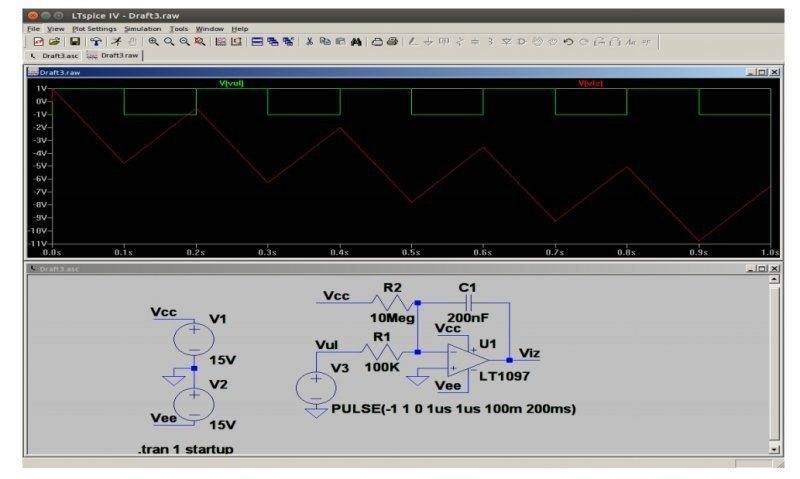Then change capacitor's value from 200nF to 33nF and output voltage will be: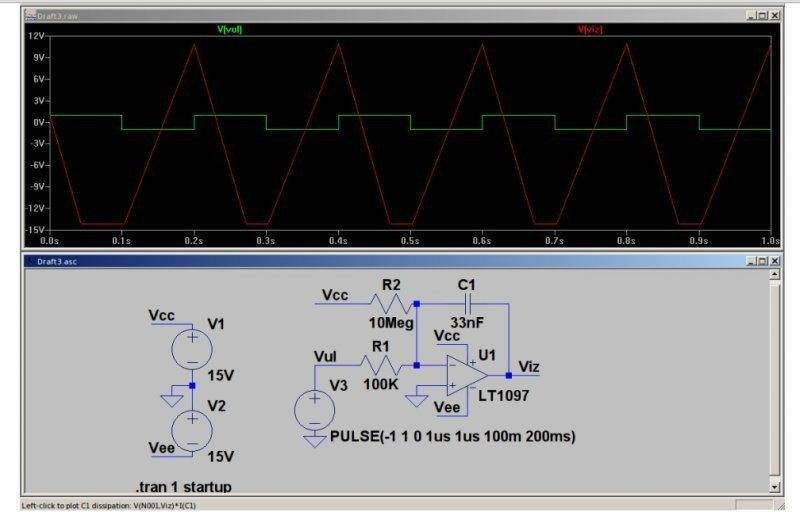Explain why output voltages are different ( first has triangle shape and gradually decreases and second has cut off bottom and doesn't decrease).

Here is what I have done:
Since there are two sources, one DC and one time dependent pulse waveform, I used superposition method. When time dependent source is active, our circuit is: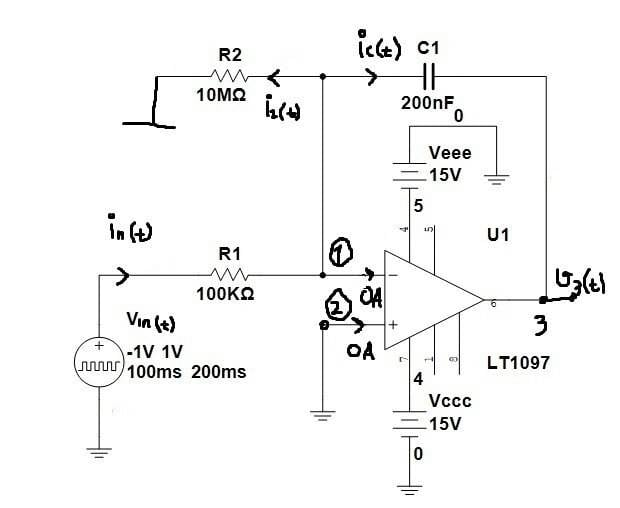$$v2(t)=0\rightarrow v1(t)=0$$

$$i2(t)=0\rightarrow iin(t)=ic(t)$$

$$iin(t)=\frac{vin(t)}{R1}$$

$$v3(t)+\frac{1}{C}\int ic(t)dt+R1iin(t)-vin(t)=0$$

For positive input voltage $$vin(t)=1V$$ we have:

$$v3(t)=-\frac{1}{RC1}\int vin(t)dt=-50\int 1dt=-50t,\,\, 0\leq t\leq 100*10^{-3}s$$

For negative input voltage $$vin(t)=-1V$$ we have:
$$v3(t)=-\frac{1}{RC1}\int vin(t)dt=-50\int (-1)dt=50t,\,\,100*10^{-3}s\leq t\leq 200*10^{-3}s$$
But I don't know what to do next :(

NascentOxygen
Staff Emeritus
The output should comprise a triangle wave produced by integrating the squarewave, plus a slow ramp produced by integrating a small fixed current. Does your mathematical derivation exhibit these two features?

The waveform from your simulation is what I'd expect to see.

Here is how I have done it:
I found Fourier series of $$vin(t)$$, $$vin(t)=\sum_{n=1}^{\infty}\frac{2(1-(-1)^{n})}{n\pi }\sin{(nw0t)}$$. Then I used superposition method to find $$viz(t)$$. When $$vin(t)$$ is active, our circuit is (I didn't show OPAMP power supply):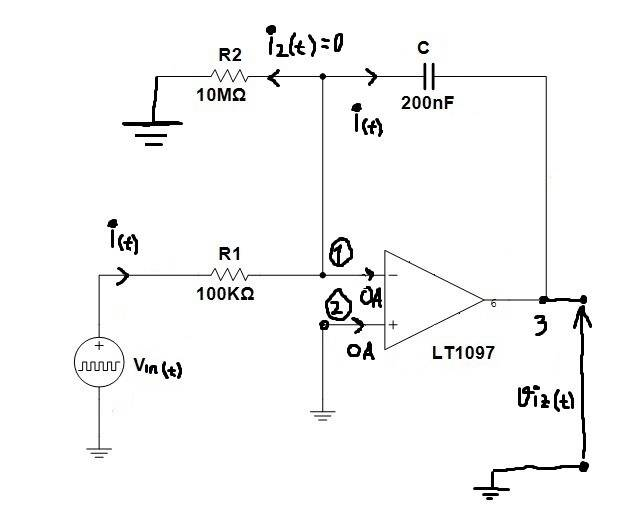Using KVL I got:
$$viz(t)+\frac{1}{C}\int i(t)+Ri(t)=vin(t)$$

$$viz(t)+\frac{1}{C}\int\frac{vin(t)}{R1}dt+Ri(t)=vin(t)$$

$$viz(t)+\frac{1}{R1C}\int vin(t)+R1\frac{vin(t)}{R1}=vin(t)$$
$$viz(t)=\frac{-1}{R1C}\int (\sum_{1}^{\infty}2\frac{1-(-1)^{n}}{n\pi }\sin{(nw0t)})dt$$
$$viz(t)=\frac{-2}{R1C}\sum_{n=1}^{\infty}\frac{1-(-1)^{n}}{n\pi }\int \sin(nw0t)dt=\frac{2}{R1C}\sum_{n=1}^{\infty}\frac{1-(-1)^{n}}{n^{2}\pi w0 }\cos{(nw0t)}$$

Here is plot of viz(t)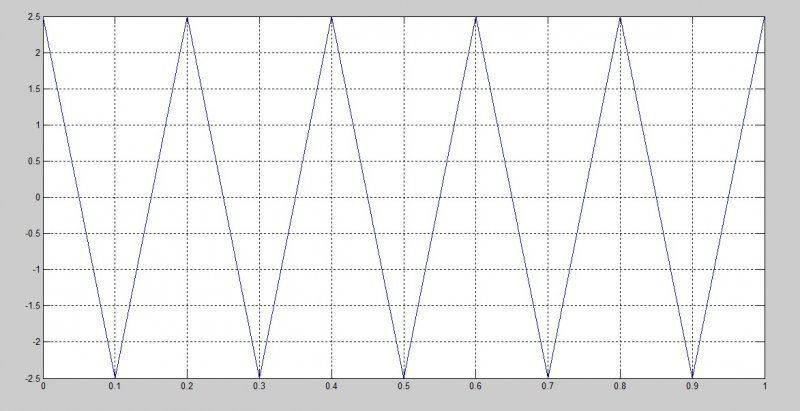Now it is necessary to do DC analysis and add viz(t) and Viz and (hopefully) we will get output as in simulator. I didn't do DC analysis yet.

Last edited:
NascentOxygen
Staff Emeritus
With referece to your infinite series... Uh huh. That's all well and good, but what does but tell you? I think you are expected to come up with an analysis and explanation of what you see in the waveforms. Along the lines of what I outlined.

OPAMP circuit and output voltage are shown in image below. Assuming that OPAMP is ideal, come up with a solution by hand.

I don't see much call for Fourier here. Not explicitly, anyway.

Last edited:
Well, I thought I must have explicit expression of input voltage for analysis. How would I express output voltage (viz(t)) if I hadn't expression for input voltage?

NascentOxygen
Staff Emeritus
Well, I thought I must have explicit expression of input voltage for analysis. How would I express output voltage (viz(t)) if I hadn't expression for input voltage?
I think you should express each input as a function of time, then integrate and sum the result.

What I think you are doing is expressing the input as a Fourier series and integrating that, then using a computer program to convert that back to a function of time and plot it. That is a roundabout process I doubt you would have time to pursue in any exam.

Have you memorised the Fourier series of a triangle wave?

Now I'm completely lost. I did analysis in Multisim and I got same shape for output voltage but different peak to peak value of signal. I give up. Here is code in Matlab I used to plot viz(t) I got by hand:
clear
clc
R1=100000;
C=200*10^(-9);
t=linspace(0,1,10000);
w0=2.*pi./0.2;
suma=0;
for n=1:10000
suma = suma + ((1-(-1).^n).*(cos(n.*w0.*t))./(n.*n.*pi.*w0));
end
red=(2./(R1.*C)).*suma;
plot(t,red)
grid

Last edited:
NascentOxygen
Staff Emeritus
In my view, you've shown all the mathematics that's needed back in your first post: the integral of a step. Now, apply that to one cycle and show how the squarewave input becomes a triangular wave at the output, and how the other input leads to a ramp at the output. Then combine them.

It might be instructive if you were to extend the duration of your simulation, so you capture some "interesting output" when non-linear things start to appear.

Before you dive into complicated maths you should look back at what you are specifically asked to explain, in post #1. I interpret it to be they want you to demonstrate a practical understanding of that circuit's behaviour under the two different conditions.

•etf
NascentOxygen
Staff Emeritus
When using the 33nF capacitor, could you also please record the waveform at the junction of R1 and R2. It might contain some answers ....

I think I solved it (without Fourier series :) )
Firstly I analysed circuit for t between 0 and 0.1s (VCC=15V, vin(t)=1V). I got vout(t)=-57.5t. Then I analysed circuit for t between 0.1s and 0.2s (VCC=15V, vin(t)=-1V). I got vout(t)=42.5t. On time interval between 0s and 0.2s our output voltage vout(t) isWhat do you think? I didn't plot it on some wider interval since it's a little bit tricky to connect those lines and plot :)

Here is code I used for plot:
>> t1=linspace(0,0.1,100000);
>> t2=linspace(0.1,0.2,100000);
>> vout1=-57.5.*t1;
>> vout2=-5.75+42.5.*(t2-0.1);
>> plot(t1,vout1,t2,vout2)
>> grid
>>
It is necessary to plot decreasing line, then on it's end increasing one etc. :)

NascentOxygen
Staff Emeritus